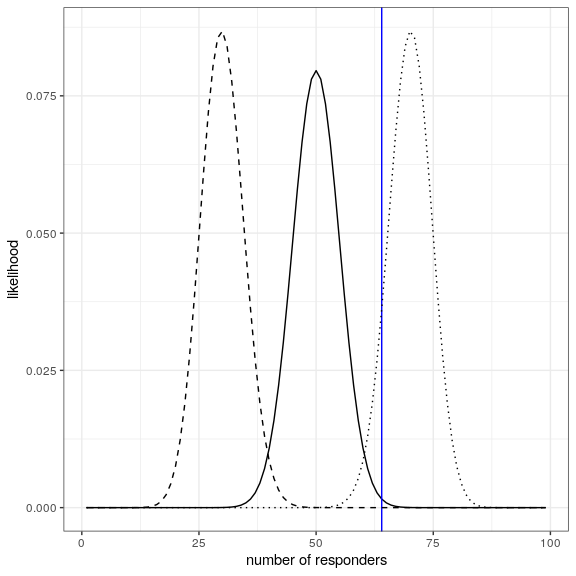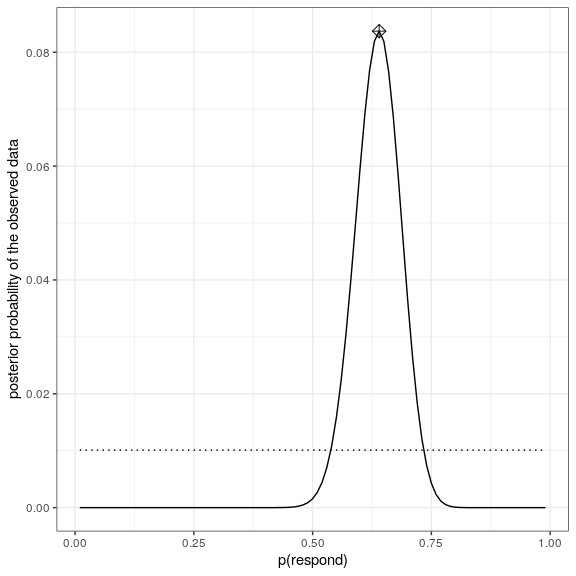# 21 Bayesian statistics in R

## 21.1 A simple example (Section 20.3)

bayes_df = data.frame(prior=NA,
likelihood=NA,
marginal_likelihood=NA,
posterior=NA)

bayes_df$prior <- 1/1000000 nTests <- 3 nPositives <- 3 sensitivity <- 0.99 specificity <- 0.99 bayes_df$likelihood <- dbinom(nPositives, nTests, 0.99)

bayes_df$marginal_likelihood <- dbinom( x = nPositives, size = nTests, prob = sensitivity ) * bayes_df$prior +
dbinom(
x = nPositives,
size = nTests,
prob = 1 - specificity
) *
(1 - bayes_df$prior) bayes_df$posterior <-
(bayes_df$likelihood * bayes_df$prior) /
bayes_df$marginal_likelihood ## 21.2 Estimating posterior distributions (Section 20.4) # create a table with results nResponders <- 64 nTested <- 100 drugDf <- tibble( outcome = c("improved", "not improved"), number = c(nResponders, nTested - nResponders) ) Computing likelihood likeDf <- tibble(resp = seq(1,99,1)) %>% mutate( presp=resp/100, likelihood5 = dbinom(resp,100,.5), likelihood7 = dbinom(resp,100,.7), likelihood3 = dbinom(resp,100,.3) ) ggplot(likeDf,aes(resp,likelihood5)) + geom_line() + xlab('number of responders') + ylab('likelihood') + geom_vline(xintercept = drugDf$number,color='blue') +
geom_line(aes(resp,likelihood7),linetype='dotted') +
geom_line(aes(resp,likelihood3),linetype='dashed')Computing marginal likelihood

# compute marginal likelihood
likeDf <-
likeDf %>%
mutate(uniform_prior = array(1 / n()))

# multiply each likelihood by prior and add them up
marginal_likelihood <-
sum(
dbinom(
x = nResponders, # the number who responded to the drug
size = 100, # the number tested
likeDf$presp # the likelihood of each response ) * likeDf$uniform_prior
)

Comuting posterior

bayesDf <-
tibble(
steps = seq(from = 0.01, to = 0.99, by = 0.01)
) %>%
mutate(
likelihoods = dbinom(
x = nResponders,
size = 100,
prob = steps
),
priors = dunif(steps) / length(steps),
posteriors = (likelihoods * priors) / marginal_likelihood
)

# compute MAP estimate
MAP_estimate <-
bayesDf %>%
arrange(desc(posteriors)) %>%
slice(1) %>%
pull(steps)

ggplot(bayesDf,aes(steps,posteriors)) +
geom_line() +
geom_line(aes(steps,priors),
color='black',
linetype='dotted') +
xlab('p(respond)') +
ylab('posterior probability of the observed data') +
annotate(
"point",
x = MAP_estimate,
y = max(bayesDf\$posteriors),
shape=9,
size = 3
)## 21.3 Bayes factors (Section 20.6.1)

Example showing how BFs and p-values relate